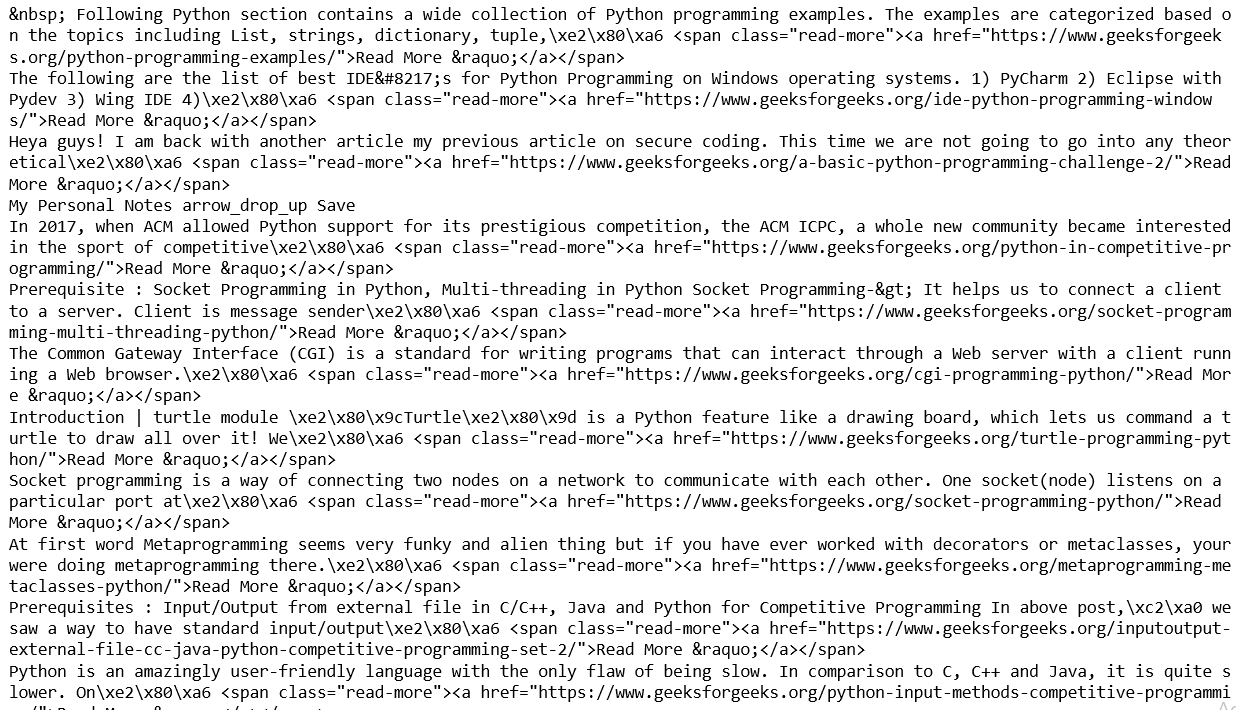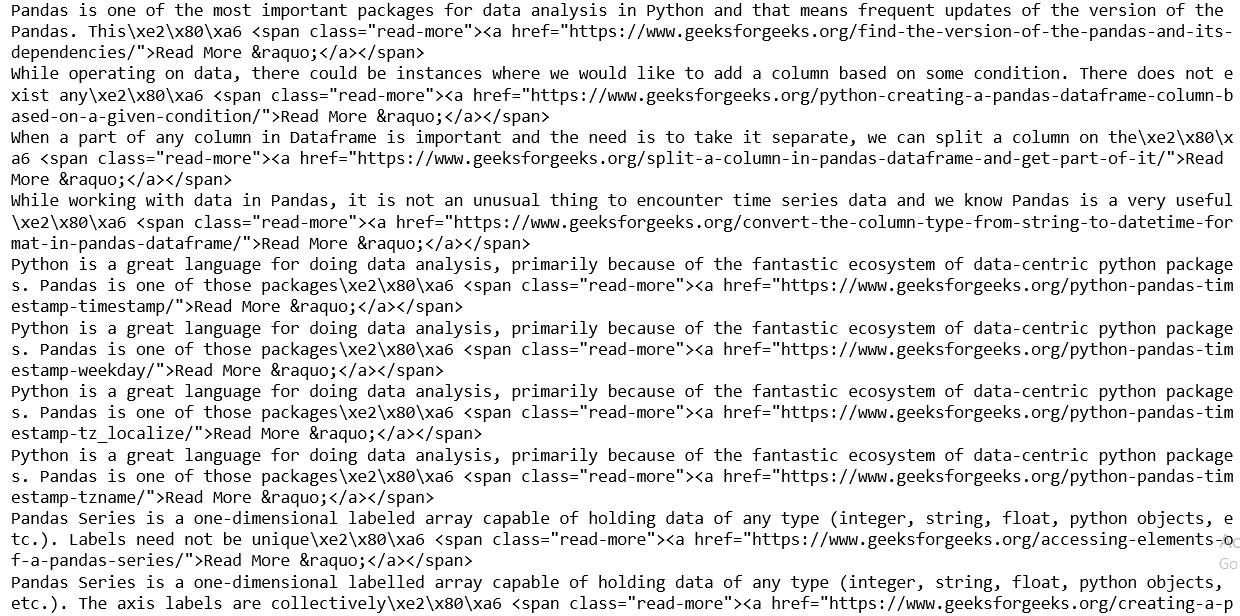# Python | Parse a website with regex and urllib

Let’s discuss the concept of parsing using python. In python we have lot of modules but for parsing we only need urllib and re i.e regular expression. By using both of these libraries we can fetch the data on web pages.

Note that parsing of websites means that fetch the whole source code and that we want to search using a given url link, it will give you the output as the bulk of HTML content that you can’t understand. Let’s see the demonstration with an explanation to let you understand more about parsing.

Code #1: Libraries needed

 `# importing libraries ` `import` `urllib.request ` `import` `urllib.parse ` `import` `re `

Code #2:

 `url ``=` `'https://www.geeksforgeeks.org/'` `values ``=` `{``'s'``:``'python programming'``, ` `          ``'submit'``:``'search'``} `

We have defined a url and some related values that we want to search. Remember that we define values as a dictionary and in this key value pair we define python programming to search on the defined url.

Code #3:

 `data ``=` `urllib.parse.urlencode(values)         ` `data ``=` `data.encode(``'utf-8'``)                   ` `req ``=` `urllib.request.Request(url, data)       ` `resp ``=` `urllib.request.urlopen(req)     ` `        `  `respData ``=` `resp.read()                       `

In the first line we encode the values that we have defined earlier, then (line 2) we encode the same data that is understand by machine.
In 3rd line of code we request for values in the defined url, then use the module `urlopen()` to open the web document that HTML.
In the last line `read()` will help read the document line by line and assign it to respData named variable.

Code #4:

 `paragraphs ``=` `re.findall(r``'

(.*?)

'``, ``str``(respData)) ` ` `  `for` `eachP ``in` `paragraphs: ` `    ``print``(eachP) `

In order to extract the relevant data we apply regular expression. Second argument must be type string and if we want to print the data we apply simple print function.

Below are few examples:

Example #1:

 `import` `urllib.request ` `import` `urllib.parse ` `import` `re ` `  `  `url ``=` `'https://www.geeksforgeeks.org/'` `values ``=` `{``'s'``:``'python programming'``, ` `          ``'submit'``:``'search'``} ` `  `  `data ``=` `urllib.parse.urlencode(values) ` `data ``=` `data.encode(``'utf-8'``) ` `req ``=` `urllib.request.Request(url, data) ` `resp ``=` `urllib.request.urlopen(req) ` `respData ``=` `resp.read() ` `  `  `paragraphs ``=` `re.findall(r``'

(.*?)

'``,``str``(respData)) ` `  `  `for` `eachP ``in` `paragraphs: ` `    ``print``(eachP) `

Output:Example #2:

 `import` `urllib.request ` `import` `urllib.parse ` `import` `re ` `  `  `url ``=` `'https://www.geeksforgeeks.org/'` `values ``=` `{``'s'``:``'pandas'``, ` `          ``'submit'``:``'search'``} ` `  `  `data ``=` `urllib.parse.urlencode(values) ` `data ``=` `data.encode(``'utf-8'``) ` `req ``=` `urllib.request.Request(url, data) ` `resp ``=` `urllib.request.urlopen(req) ` `respData ``=` `resp.read() ` `  `  `paragraphs ``=` `re.findall(r``'

(.*?)

'``,``str``(respData)) ` `  `  `for` `eachP ``in` `paragraphs: ` `    ``print``(eachP) `

Output:My Personal Notes arrow_drop_upCheck out this Author's contributed articles.

If you like GeeksforGeeks and would like to contribute, you can also write an article using contribute.geeksforgeeks.org or mail your article to contribute@geeksforgeeks.org. See your article appearing on the GeeksforGeeks main page and help other Geeks.

Please Improve this article if you find anything incorrect by clicking on the "Improve Article" button below.

Article Tags :

2

Please write to us at contribute@geeksforgeeks.org to report any issue with the above content.